# Earth Curvature Simulation by Walter Bislins

#### Mick West

Staff member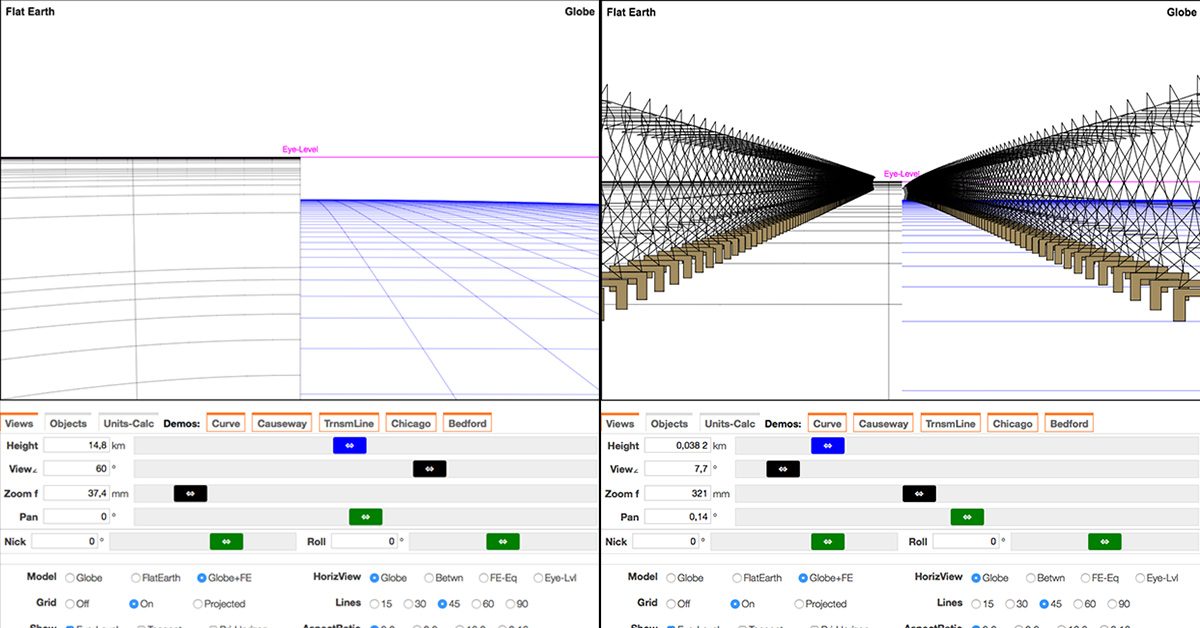Walter Bislins has created a very useful tool for visualizing what things should look like from certain positions, and with certain camera settings, on the Globe Earth, or on a hypothetical Flat Earth.

The Simulator includes recreations of classic and modern experiments and observations including the Bedford Level, the Pontchartrain Power Lines and Causeway, and the Chicago Skyline. You can stop the simulation at any point, and examine the scene and the settings used. Here's a (non-interactive) animation of the Bedford Level experiment.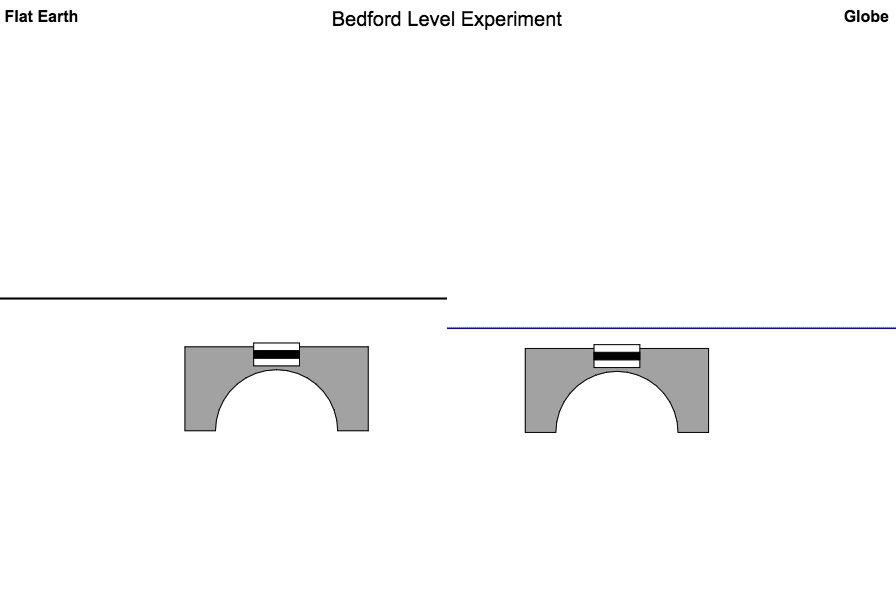I'd encourage anyone interested in the topic to play around with the various settings and watch all five demos.

Simulator with discussion:
http://walter.bislins.ch/bloge/index.asp?page=Flat-Earth:+Finding+the+curvature+of+the+Earth
http://walter.bislins.ch/blog/index...h%3A+Wie+stark+ist+die+Kr%FCmmung+der+Erde%3F

Simulator alone:
http://walter.bislins.ch/blog/index.asp?page=Earth+Curvature+Simulation

Last edited:

#### Antrax

##### New Member
I've used this simulator for high altitude footage I found on Reddit/Youtube, which according to the author was taken at an altitude of 115,000feet/35km with a Canon Powershot SD880 set to 35mm film equivalent of f=40mm.
I'm not really good with photoshop and had to do it on my smartphone, so it doesn't look as good as the examples made by Walter. Anyway, here's what I got:Last edited by a moderator:

#### Enricks

##### Member
So in the Bedford Level experiment the middle pole was totally visible, but below the line of sight and above the distant mark?

#### Mick West

Staff member
So in the Bedford Level experiment the middle pole was totally visible, but below the line of sight and above the distant mark?

"Line of sight" can refer to any line, but I think you mean "eye level" - i.e. a horizontal line from the telescope, perpendicular to the vertical direction at the telescope?

From any given position the water surface is going to seem to "drop" away from the horizontal plane (it's like you are standing on top of a very large ball). So the middle pole is going to be below that plane, and the distant bridge will be below that.

So if you take a line of sight from the telescope to the distant bridge, then the middle marker will appear above that.

#### Enricks

##### Member
"Line of sight" can refer to any line, but I think you mean "eye level" - i.e. a horizontal line from the telescope, perpendicular to the vertical direction at the telescope?

From any given position the water surface is going to seem to "drop" away from the horizontal plane (it's like you are standing on top of a very large ball). So the middle pole is going to be below that plane, and the distant bridge will be below that.

So if you take a line of sight from the telescope to the distant bridge, then the middle marker will appear above that.

Yes, sorry for my slip of tongue there: I meant eye level. I found that odd because at sea level the "drop" should be 0, like you showed in your "horizon dip" demonstration, so both the horizon and the pole should not show any drop. I don't know if I'm misunderstanding something (almost certainly yes lmao).

#### Mick West

Staff member
Yes, sorry for my slip of tongue there: I meant eye level. I found that odd because at sea level the "drop" should be 0, like you showed in your "horizon dip" demonstration, so both the horizon and the pole should not show any drop. I don't know if I'm misunderstanding something (almost certainly yes lmao).

The side to side visual "drop" of the horizon is zero at sea level. But that's a totally different thing to the curve of the Earth away from you.

And here the telescope is not at the water level. It's like 12 feet above the water, looking at things that are 3 and six miles away. The thing 3 miles away will be 6 feet below the horizontal plane passing through the telescope, and the thing 6 miles away will be 24 feet below that plane.

The key thing though is the "bulge", which is 6 feet over 6 miles. i.e. the middle marker will be six feet higher than a line of sight between the end points (assuming all are the same height above the water)

#### Enricks

##### Member
I asked this because in the animation you provided above, the horizon seems to be dropping below eye-level (i.e. the crosshair), but maybe I'm making a mistake somewhere.

#### Mick West

Staff member
I asked this because in the animation you provided above, the horizon seems to be dropping below eye-level (i.e. the crosshair), but maybe I'm making a mistake somewhere.

No that's correct. The horizon is always below eye level if your eye is above the water level. Not a lot when you are close to the water level, but the effect is magnified here because of the high zoom.

#### Rory

##### Closed Account
So in the Bedford Level experiment the middle pole was totally visible, but below [eye level] and above the distant mark?
I found that odd because at sea level the "drop" should be 0, like you showed in your "horizon dip" demonstration, so both the horizon and the pole should not show any drop. I don't know if I'm misunderstanding something.
I'm not quite sure what you mean by "drop"? Or why it should be zero?

Also, to clarify, it's not so much about measurements at "sea level" - for example, doing calculations for a view across a high elevation mountain lake, you'd want to know the height above the water, rather than above sea level.

Though I think you get that already; thought I'd mention it just in case.

#### Enricks

##### Member
I'm not quite sure what you mean by "drop"? Or why it should be zero?

Also, to clarify, it's not so much about measurements at "sea level" - for example, doing calculations for a view across a high elevation mountain lake, you'd want to know the height above the water, rather than above sea level.

Though I think you get that already; thought I'd mention it just in case.

Never mind, Mick already gave me a satisfying answerEDIT: btw, by "drop" I meant horizon dip.

Last edited:

#### Pertti Niukkanen

##### Member
Walter Bislin's calculator "Finding the curvature of the Earth" can also tell "the left-right drop angle δ" (the side to side visual "drop" of the horizon). http://walter.bislins.ch/bloge/index.asp?page=Finding+the+curvature+of+the+Earth. Is this value in Mick West's calculator too? I couldn’t find it.

I put in the Bislin's calculator the following values (picture below):
Height: 1000 m
Field of view: 60 degrees
Koeff. k = 0 (no refraction)
The calculator gives the Left-Right Drop Angle = 0.1046 degrees.

For a derivation of how Bislin's HorLftRgt values are calculated see "Calculating left-right Horizon Drop", http://walter.bislins.ch/bloge/index.asp?page=Calculating+left-right+Horizon+Drop.

I tried to use Bislin's equations putting them in Excel. Something must be wrong, because I got not the same values as Bislin.

Here is comparison of the "left-right drop angle δ" in different heights when field of view is 60 degrees and refraction is not accounted. In the first column is the height (m), in the second the angle δ in degrees according to Bislin's calculator and in the third column is the angle δ which I got using Bislin's equations.

The last column shows that my values are always about 1.5 times larger than the values the calculator gives.

2 –––––––– 0.004678 ––– 0.007023 ––– 1.5013
10 ––––––– 0.01046 –––– 0.01571 –––– 1.5014
100 –––––– 0.03308 –––– 0.04966 –––– 1.5013
1000 ––––– 0.1046 ––––– 0.1571 ––––– 1.5015
10000 –––– 0.3309 ––––– 0.4969 ––––– 1.5018
100000 ––– 1.052 –––––– 1.5807 ––––– 1.5026

Maybe I do not know how to use the equations Bislin gave. Would somebody please calculate the angle δ with Bislin's formulas (in situation like below)? Is the angle 0.1046 degrees (like in the picture) or 0.1571 degrees (like I got with the formulas)? Or maybe neither?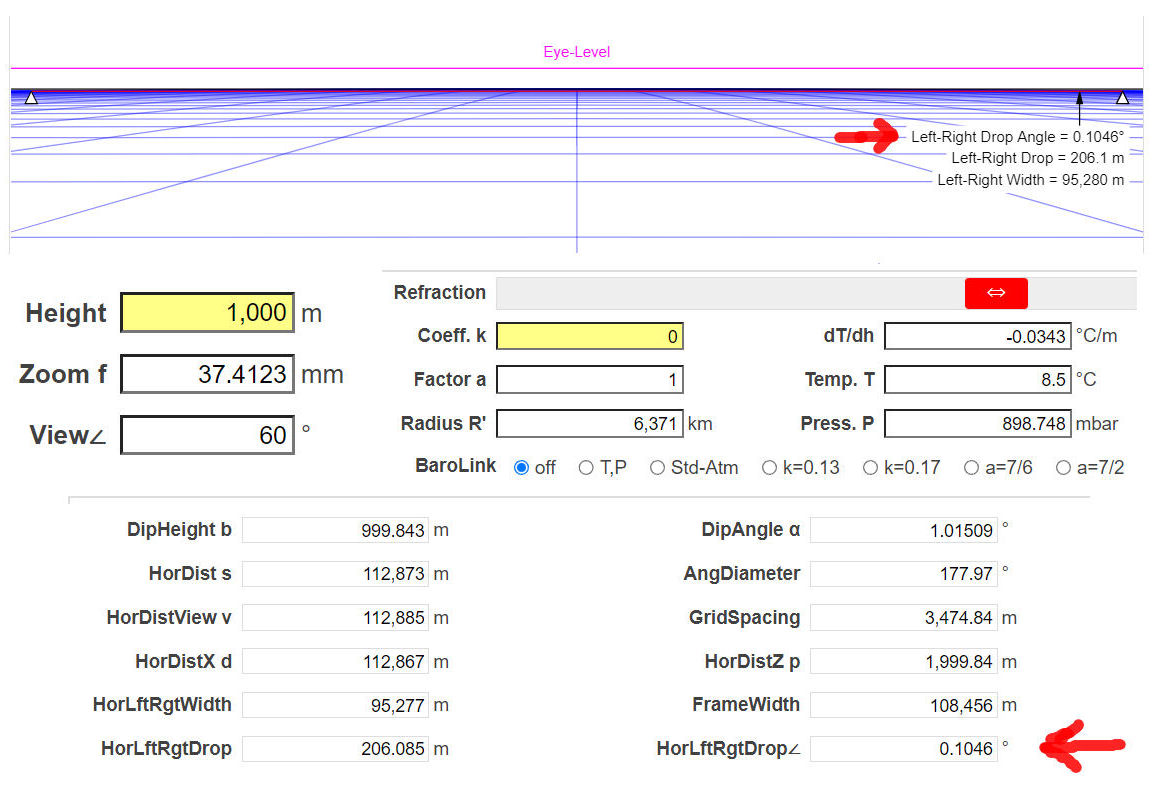#### Mick West

Staff member
Walter Bislin's calculator "Finding the curvature of the Earth" can also tell "the left-right drop angle δ" (the side to side visual "drop" of the horizon). http://walter.bislins.ch/bloge/index.asp?page=Finding+the+curvature+of+the+Earth. Is this value in Mick West's calculator too? I couldn’t find it.
Yes, you need to used the "advanced" check box, or:
https://www.metabunk.org/curve/?d=6&h=1000&r=6371&u=m&a=a&fd=60&fp=3264

These numbers are notoriously tricky, as you need to be very clear about exactly what you are calculating and ensure you're using the same underlying terminology and constants. For a detailed discussion of some of the issues, see:

Generally, you are concerned with how things look in photos, so really calculating it in pixels (or % of the height of the image) is the most practical end result.

#### Mendel

##### Senior Member.
The last column shows that my values are always about 1.5 times larger than the values the calculator gives.
Bislins distinguishes between diagonal FOV and horizontal FOV, mixing these up may be a source of error?

#### Pertti Niukkanen

##### Member
Thank you for your answers Mick and Mendel. I realized that these numbers really are tricky.

I took Bislin's derivation for the "left-right drop angle δ" as purely a geometrical calculation. I found there no error. His equations give the correct answer to this geometrical problem. I'm also quite sure, that my Excel-spreadsheet uses those equations correctly so that I can depend on it.

I got the angle δ = 0.15705573 degrees (height 1000 m, field of view 60 degrees, no refraction). Your calculator gives Horizon Curve Angle v2 = 0.15705. The values are practically the same. This is true up to height 100 km (at least). So the angle δ in my Excel-spreadsheet (and in Bislin's formulas) is the same as your angle v2? (I wouldn't ask what calculations you used.)

Why is it then, that in Bislin's picture the "left-right drop angle" = 0.1046 degrees? His formulas (and my Excel and yours v2) give 1.5 times greater value 0.15706. You must glide the high to 2252 m to get the same angle 0.15706. The difference is not great in the pictures below but yet noticeable.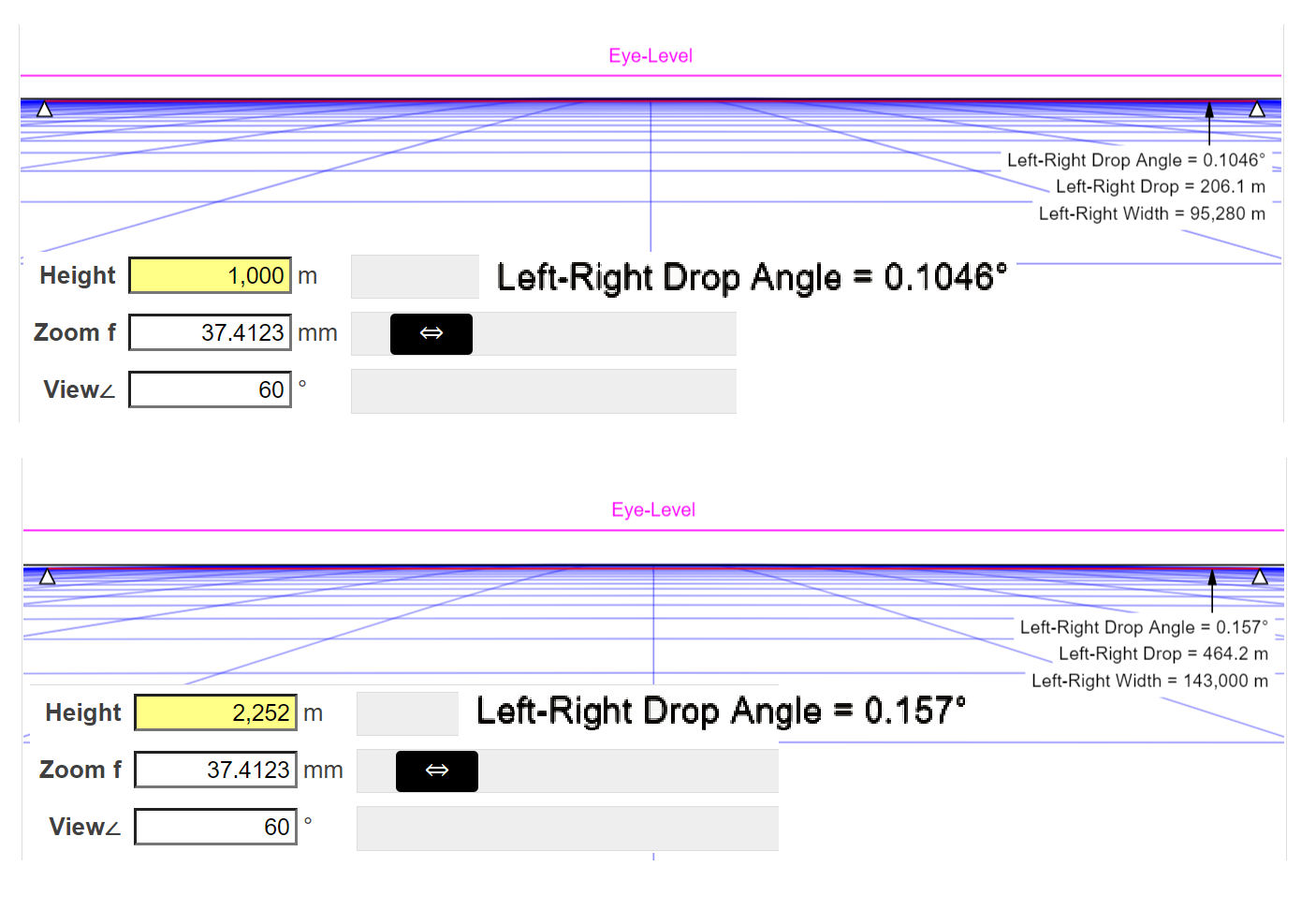I'm sure that the angle values and simulation pictures in Bislin's calculator are the best way to represent the situation. Cause of the difference might be what Mendel mentioned. This topic just came to my mind when I saw FE-memes like this:I planned to make a table by Excel, showing the height of the observer with the corresponding left-right drop angle δ. So I could ask the flat-Earthers: "Do you think you can notice the horizons curve with a bulge of 0.15706 degrees from the height of 1000 m?" If I use screenshots from Bislin's calculator as illustration, I must ask:"Do you think you can see a curve of 0.1046 degrees from the height of 1000 m?"

Well, I can easily fit my Excel-values in Bislin's pictures by dividing them by 1.5. So I get easily a long and impressive table with illustrations to stupefy the FE-people. I really don’t like living on an empty kitchen paper roll. Do you think this much "cheat" is acceptable?#### Pertti Niukkanen

##### Member
Yes, you need to used the "advanced" check box, or:
https://www.metabunk.org/curve/?d=6&h=1000&r=6371&u=m&a=a&fd=60&fp=3264

These numbers are notoriously tricky, as you need to be very clear about exactly what you are calculating and ensure you're using the same underlying terminology and constants. For a detailed discussion of some of the issues, see:

Generally, you are concerned with how things look in photos, so really calculating it in pixels (or % of the height of the image) is the most practical end result.

In an interview Neil deGrasse Tyson said about Felix Baumgartner's 39 km jump: "At that height you don't see the curvature of the earth. That stuff is flat!" (Watch the video from 38:20 on) Source: https://www.youtube.com/watch?v=0FMGTVCIDbU&t=2300s

In the context of the interview, it's obvious what Tyson was trying to say. Of course the flat-earthers took his phrase "That stuff is flat!" literally repeating it gleefully. (Read also the conversation here: https://www.metabunk.org/threads/debunked-neil-degrasse-tyson-that-stuff-is-flat.10723/. Mick West commented there: But on the face of it his [Tyson] assertion that "you don't see the curvature of the earth" from 128,000 feet is just flat wrong.)

Should Tyson said: "At that height you don't see the curvature because it is so small." Or being more specific: "If the field of view is 60 degrees, the left-right drop angle is only xxx degrees." But how large is this angle xxx?

When you look at the horizon and your field of view is, let us say, 60 degrees, you see a circular segment ⌓. The height of this segment is called sagitta (or bulge). So we can ask, at which angle you see this sagitta (or what is the sagitta's angular size)?

This question has bothered me a long time. So I was happy to find the answer by Walter Bislin: http://walter.bislins.ch/bloge/index.asp?page=Calculating+left-right+Horizon+Drop. It's purely a geometric problem to find the angle δ when R, h and α are known. Below is Bislin's general solution put together as one formula (1).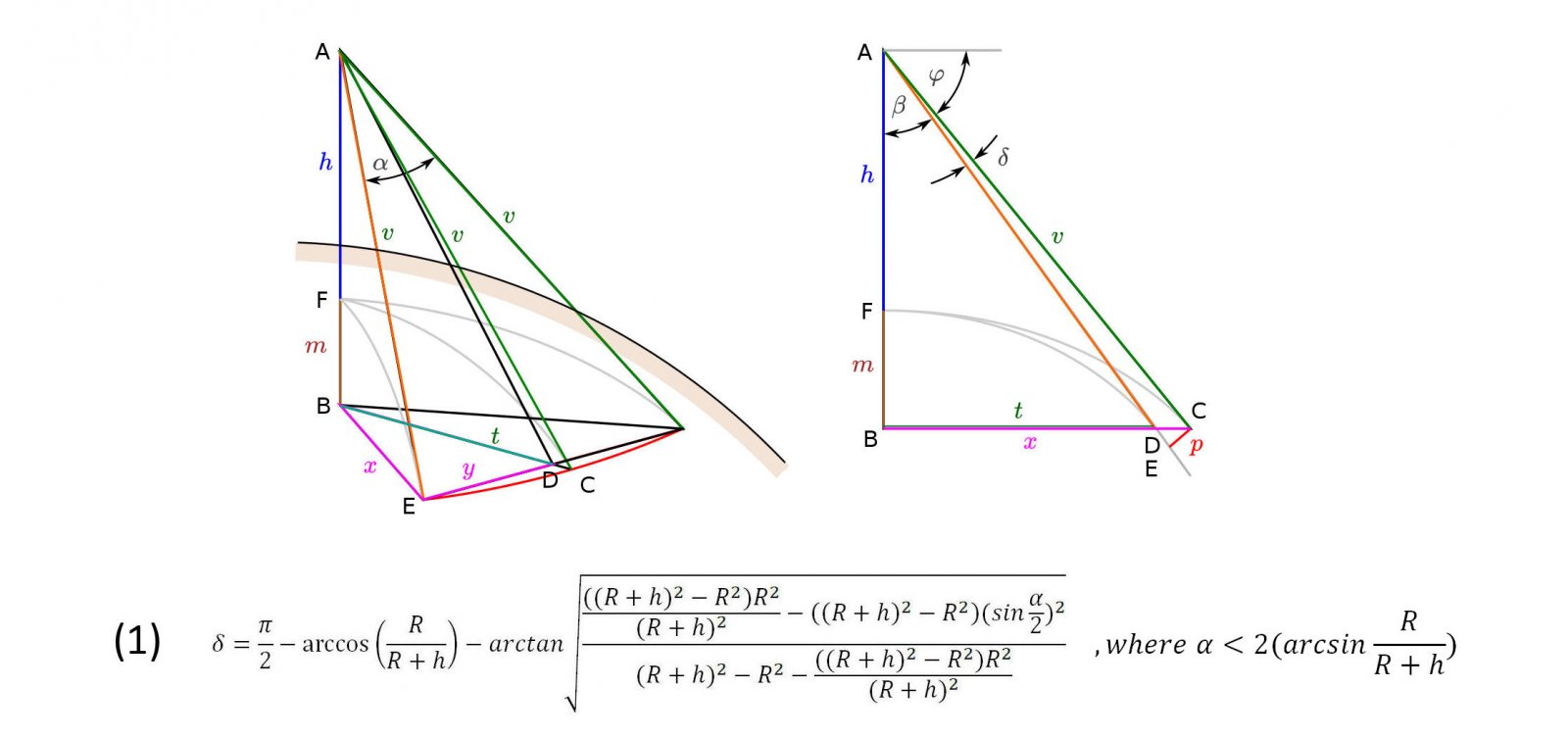Here you get the angle δ in radians. Transform it to degrees by multiplying by 180/π.

The inequality in the "under the condition that"-expression means, that the field of view must not be too large. Otherwise the sides of the viewing angle will not touch the ball at all. The sketches below try to clarify this idea in the case where h = R. Note that the views are still in 3D. So the field of view angle α might look a little bizarre. Here δ is the angle at which the sagitta s is seen from point A. The values of δ are calculated with the formula (1).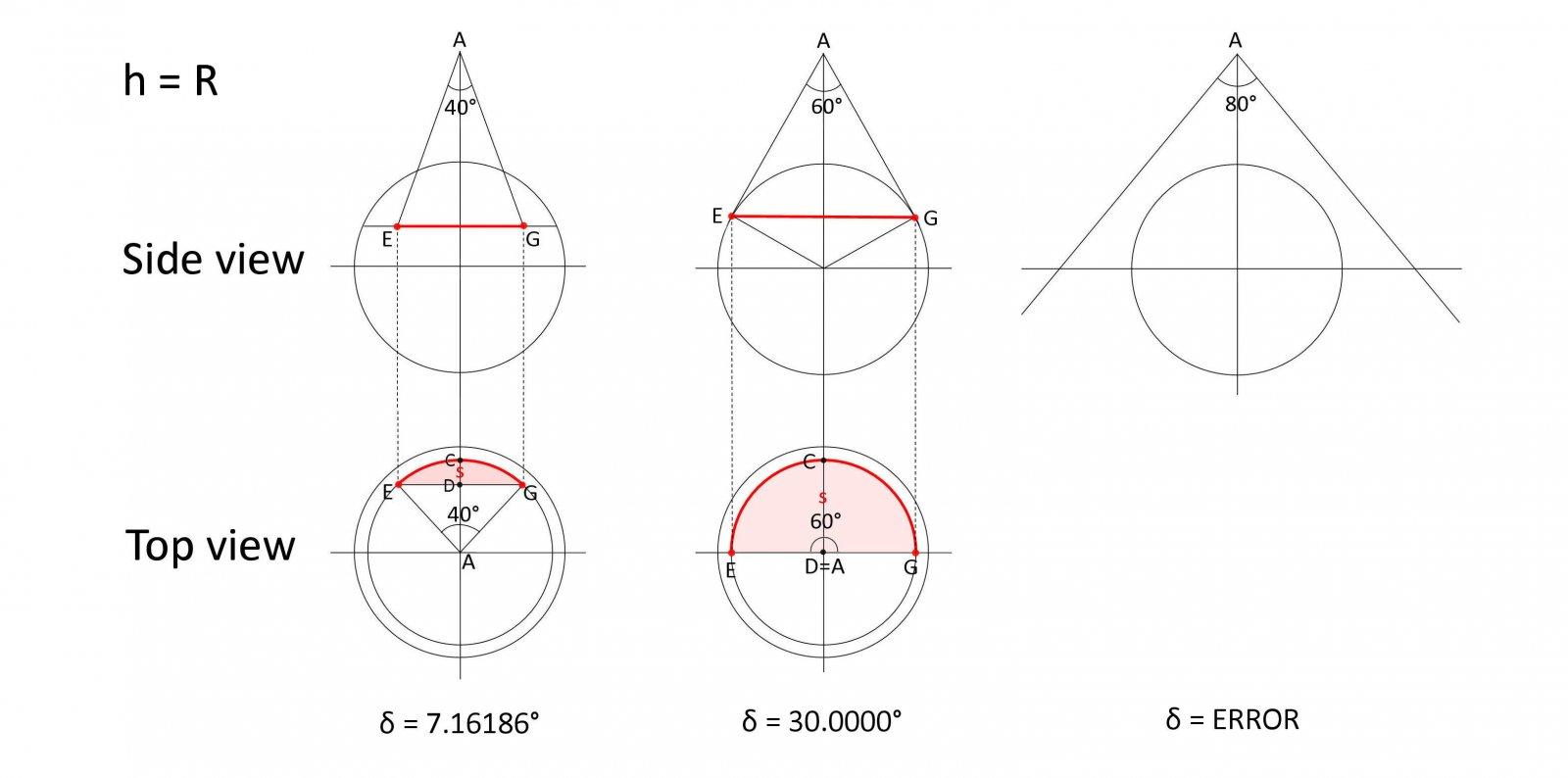So this is the answer I've been looking for. Now I can say in the case of Felix Baumgartner's 39 km jump:
If your field of view is 60 degrees, the left-right drop angle δ is only 0.98323 degrees. So the curvature of the horizon is really hard to see without proper measurements.

But is this the right answer after all?

Walter Bislin's calculator gives HorLftRgtDrop∠ = 0.65462°. It's about 2/3 of the value of δ which is 0.98323°. The difference is remarkable. Certainly there is a reason for this difference, but Bislin doesn’t tell what it is.

Metabunk calculator gives corresponding information: "Horizon Curve refers to how much the left and right ends of the horizon are vertically lower than the middle of the image, measured in various ways. Horizon Curve Angle = Horizon Curve height expresses as a visual angle. There are two ways of calculating this which are very similar for most heights."

In the case of Felix Baumgartner's 39 km jump these values are:
– Horizon Curve Angle v1= 0.96842
– Horizon Curve Angle v2 = 0.98215

Especially v2 is at small heights almost the same as δ. In bigger heights it differs more though.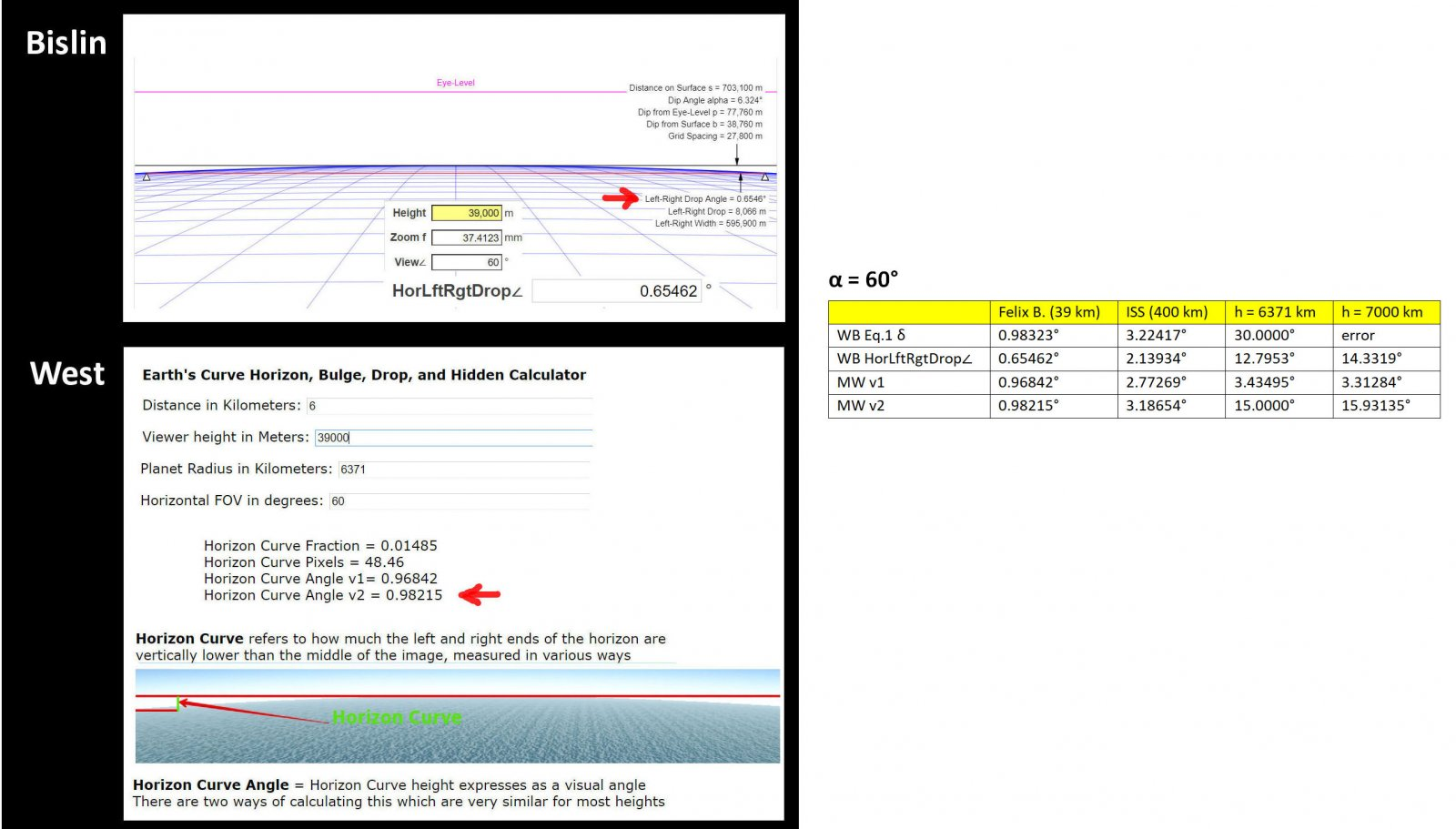So neither calculator gives the value δ = 0.98323. Maybe the values that calculators give are more useful in analysing photographs. I really know nothing of that topic. Maybe Mick could clarify this topic a little bit? And could this pure geometric value δ be added in the calculator as reference like v0?

I would be very happy with δ. I still think it's the best and unambiguous answer to the question "How big was the curve that Baumgartner saw?" Am I wrong?

Moreover I have a worry on my mind. If some pedantic flat-earther checks the angular size of the sagitta in Metabunk and Bislin calculators, he may find three (or four) different values. That's where the cry begins: THEY even contradict themselves!Last edited:

#### Pertti Niukkanen

##### Member
I put formula (1) in Excel. Now it's easy to change input values R, α and h and get the angle δ in all cases. The figures of R and h must be proportional (in the same unit or no unit). Angle α must be fed in degrees.

Select the cell D2 and type the formula

=180/PII()*(PII()/2-ACOS(A2/(A2+C2))-ATAN(NELIÖJUURI(((NELIÖJUURI(((A2+C2)^2-A2^2))*A2)/(A2+C2))^2-(NELIÖJUURI(((A2+C2)^2-A2^2))*SIN((B2*PII()/180)/2))^2)/(NELIÖJUURI((NELIÖJUURI(((A2+C2)^2-A2^2)))^2-((NELIÖJUURI(((A2+C2)^2-A2^2))*A2)/(A2+C2))^2)-C2+C2)))

This formula works in Finnish version of Excel. In English version some replacements must be done:
PII() -> PI()
NELIÖJUURI -> SQRT
I guess there are no others?

Note that the decimal separator in Finnish version of Excel is comma.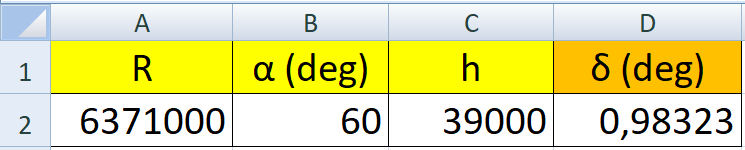Last edited:

#### Rory

##### Closed Account
Should deGrasse Tyson have said: "At that height you don't see the curvature because it is so small"?

Nope, definitely not.

Here's a photo of the curvature from the cockpit of a plane at 46,000 feet: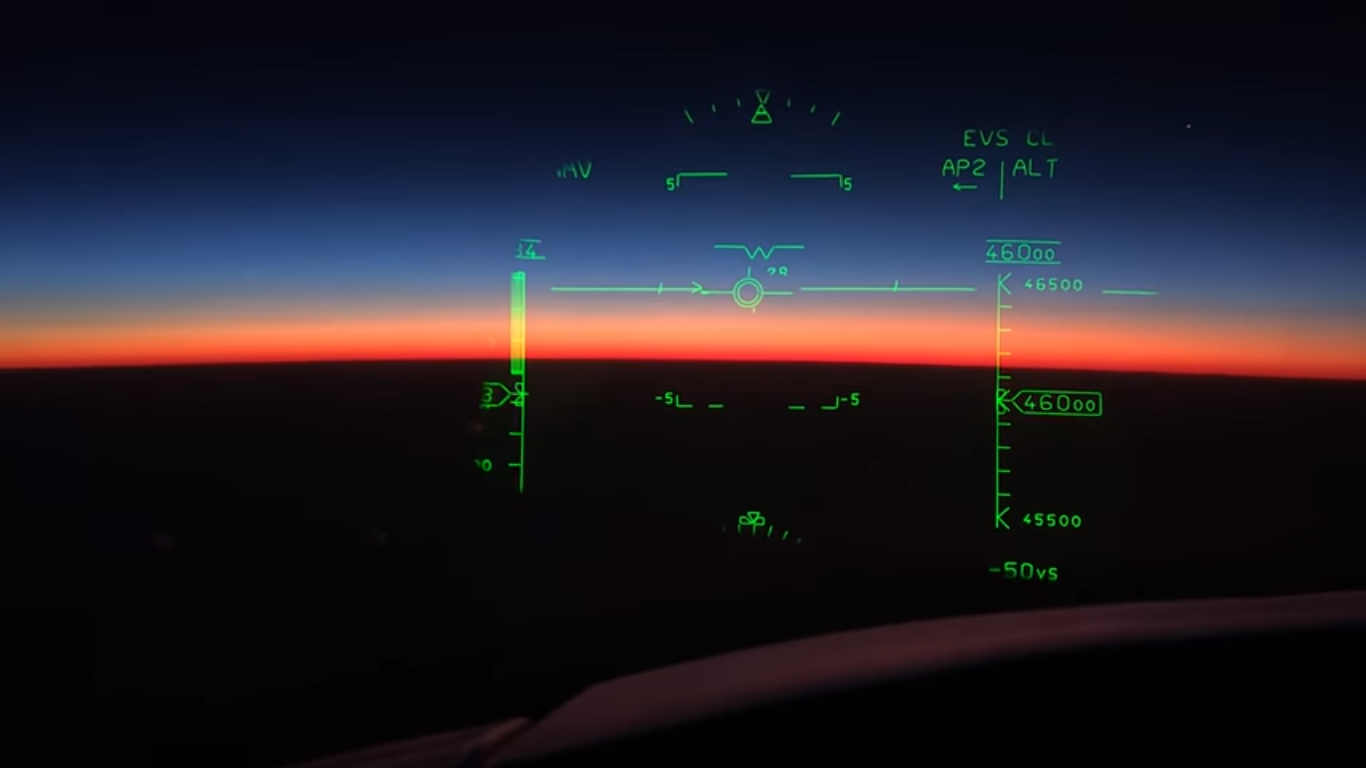"Just flat wrong" is exactly right. Sometimes deGrasse Tyson's statements are just kind of idiotic.

#### Mendel

##### Senior Member.
If your field of view is 60 degrees
horizontal 60⁰, or diagonal 60⁰?

#### FatPhil

##### Senior Member.Given that everything relevant is dimensionless ratios, you could just scale it dowm to something that you could gaze upon. E.g. the radius of the outside of a 400m running track is ~46m. Scaling the earth down to that size puts Felix's jump at just over 28cm. Maybe you could set up a few poles or sticks around the curve, put your head at the right distance and see if they appreciably bend away (spoiler - they should do - I trust you'll also put poles out for a flat-earth model too and compare the two views). Alas, that's a drop-of-horizon simulation, not a bend-of-horizon one. That would require more thought. I suspect a 0.28cm high vista of a 46cm beach-ball might be hard to get to work.

#### Mick West

Staff member
So neither calculator gives the value δ = 0.98323. Maybe the values that calculators give are more useful in analysing photographs. I really know nothing of that topic. Maybe Mick could clarify this topic a little bit? And could this pure geometric value δ be added in the calculator as reference like v0?
The values in the calculator should be what you get out of a rectilinear camera with the horizon centered. The actual code is:
JavaScript:
``````        // For horizon curve, via:
// https://www.metabunk.org/are-lynchs-horizon-calculations-correct.t7877/#post-189546
// Intermediate forms
// Z=(R*sqrt((R+h)^2-R^2))/(R+h))
// H=(((R+h)^2-R^2)/(R+h))
// ratio = SQRT(h*(h+2*R))/R*tan(a/4)/2
// angle = (atan(Z/H)-atan(Z*cos(a/2)/H))

var dropPixels = dropFraction*fovPixels;
var Z = (r*Math.sqrt((r+h)*(r+h)-r*r))/(r+h);
var H = (((r+h)*(r+h)-r*r)/(r+h));

// Second method via: https://www.metabunk.org/are-lynchs-horizon-calculations-correct.t7877/#post-189661

One essentially calculates the drop in pixel, then scales that to an angle based on a linear relationship between pixels and angle (which is not 100% accurate, as this varies across the image.
The other corrects for this across the image (left-right), as discussed here:

It has been quite some time since I looked at this (in 2016) and I might be missing (or have missed) something.

#### Mick West

Staff member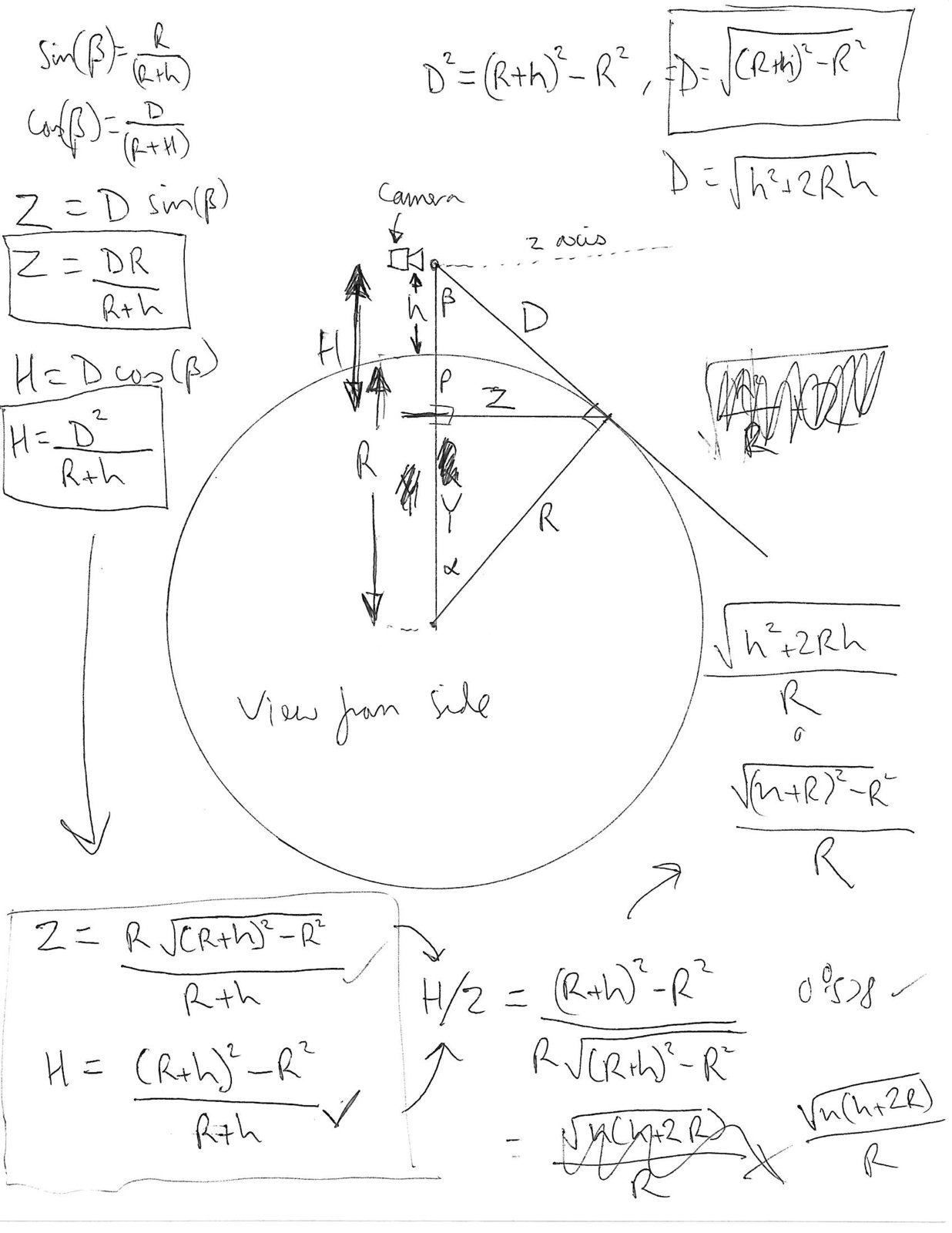My working (for Z and H) at the time (which you might have missed, as it's in a spoiler tag)

(That cos(beta) should be D/(R+h), not D/(R+H), but is used correctly when the H = (D^2)/(R+h)

Again, it's been a while and I'm not super motivated to revisit it.

#### FatPhil

##### Senior Member.
why? curvature is nonlinear

Which bit of "it scales" do you disagree with? Which bit of the opposite r adjecent do you think that you can scale without scaling the hypotenuse?

And why the -1 moderation?

#### Mendel

##### Senior Member.

Which bit of "it scales" do you disagree with? Which bit of the opposite r adjecent do you think that you can scale without scaling the hypotenuse?

And why the -1 moderation?

you need to mention that you're flipping the vertical jump into a horizontal displacement (the observer needs to be outside the track), at which point you've lost most of your audience who tends to not be good at spatial thinking

it's a red herring

#### FatPhil

##### Senior Member.

you need to mention that you're flipping the vertical jump into a horizontal displacement (the observer needs to be outside the track), at which point you've lost most of your audience who tends to not be good at spatial thinking

it's a red herring

I need to specify that the radial direction in the example corresponds to the radial direction in the thing it's trying to model?!?!

#### Pertti Niukkanen

##### Member
Given that everything relevant is dimensionless ratios, you could just scale it dowm to something that you could gaze upon. E.g. the radius of the outside of a 400m running track is ~46m. Scaling the earth down to that size puts Felix's jump at just over 28cm. Maybe you could set up a few poles or sticks around the curve, put your head at the right distance and see if they appreciably bend away (spoiler - they should do - I trust you'll also put poles out for a flat-earth model too and compare the two views). Alas, that's a drop-of-horizon simulation, not a bend-of-horizon one. That would require more thought. I suspect a 0.28cm high vista of a 46cm beach-ball might be hard to get to work.

Must say, I'm pretty much lost in this conversation by Mendel and FatPhil. It is (partly at least) because of English is not my native language. Here is how I understood FatPhil's scaling example.

FatPhil: "The radius of the outside of a 400m running track is ~46m."

I guess this circle represents the horizon circle that Baumgartner can see. Its radius is HorDistX d = 701.721 km (look http://walter.bislins.ch/bloge/index.asp?page=Finding+the+curvature+of+the+Earth).

So the scale of this "Miniearth" is about 1:15255. There
– Miniearth radius = 417.64 m
– Mini Baumgartner's height from Miniearh's surface = 2.5566 m
– Mini Baumgartner's height from the stadium surface = 5.1131 m (it's the double, just believe me)

FatPhil: "Maybe you could set up a few poles or sticks around the curve, put your head at the right distance and see if they appreciably bend away."

So your eye (close the other) is in the middle of a circle (radius = 46 m) at the height of 5.113 m above the surface of the stadium.

How should I "set up a few poles or sticks around the curve"?

I understand this so, that I set up two poles at the points on the circumference of the circle so that your eye can see these points in an angle of 60 degrees. To correspond Baumgartner's situation the poles must point to "Miniearht's" center, which is below you in the depth of 415.08 m from the surface of the stadium. So the poles are certainly not parallel or perpendicular to the surface of the stadium.

It's a bit laborious to calculate the angle between the poles, but I got the value about 6.4 degrees. This is the angle at which also your eye sees the poles.

FatPhil: "see if they appreciably bend away (spoiler - they should do)"

I am not sure, what you mean by that. But if you mean by "bend away" the angle between the poles they really do bend. Otherwise I'm still lost in this conversation.The angles are preserved in all scales. So the value δ = 0.98323 is here too. And so it is in the case of the beach ball also.

#### Mendel

##### Senior Member.
I need to specify that the radial direction in the example corresponds to the radial direction in the thing it's trying to model?!?!
I first thought you were trying to recreate the horizon (as did Pertti)

#### Mendel

##### Senior Member.
Here is how I understood FatPhil's scaling example
That's how I understood it at first, too, but no.

Phil puts the slice of Earth on the side. The outside border of the racetrack is the Earth surface, and "simulated Felix" needs to be 28 cm outside that border, to see the "Earth" "drop away" horizontally (!) when they look left and right.

It's completely offtopic to the horizon curvature discussed in this thread; you can't see that with Phil's racetrack.

#### Pertti Niukkanen

##### Member
That's how I understood it at first, too, but no.

Phil puts the slice of Earth on the side. The outside border of the racetrack is the Earth surface, and "simulated Felix" needs to be 28 cm outside that border, to see the "Earth" "drop away" horizontally (!) when they look left and right.

It's completely offtopic to the horizon curvature discussed in this thread; you can't see that with Phil's racetrack.

Thank you for the clarification. I'm happy to hear I'm not the only one who misunderstands Phil's example. And happy too that it's offtopic so I need not ponder it anymore.#### FatPhil

##### Senior Member.
That's how I understood it at first, too, but no.

Phil puts the slice of Earth on the side. The outside border of the racetrack is the Earth surface, and "simulated Felix" needs to be 28 cm outside that border, to see the "Earth" "drop away" horizontally (!) when they look left and right.

It's completely offtopic to the horizon curvature discussed in this thread; you can't see that with Phil's racetrack.

And yet Mick can see it on a piece of paper? The curve on the paper is the curve of the track. h is 28cm. The marked right angle is the outermost point of the track.
And whether it's the same horizon curvature discussed in this thread, I refer you to the image in the first post to the thread.

#### Pertti Niukkanen

##### Member
Not sure how a racetrack fits with Bislin's 3D calculator, either.

What FatPhil might mean by saying "The curve on the paper is the curve of the track. h is 28cm."

The height 28 cm is to scale with Earth's radius of 46 m.So the curve on the paper is the curve of the horizon circle when h = 28 cm and R = 4600 cm.

In Walter Bislin's Advanced Earth Curvature Calculator you can change the radius R of the Earth (minimum 100 km), http://walter.bislins.ch/bloge/index.asp?page=Advanced+Earth+Curvature+Calculator.

When I put the values h = 28000 m and R = 4600 km, I got the Left-Right Drop Angle = 0.652763°. In the corresponding Baumgartner's jump the values are: h = 39000 km and R = 6371 km. In that case I got the Left-Right Drop Angle = 0.654620°

These values are practically the same (and only 2/3 of the value of my geometric formula (1) but I understand this is no error in neither formula).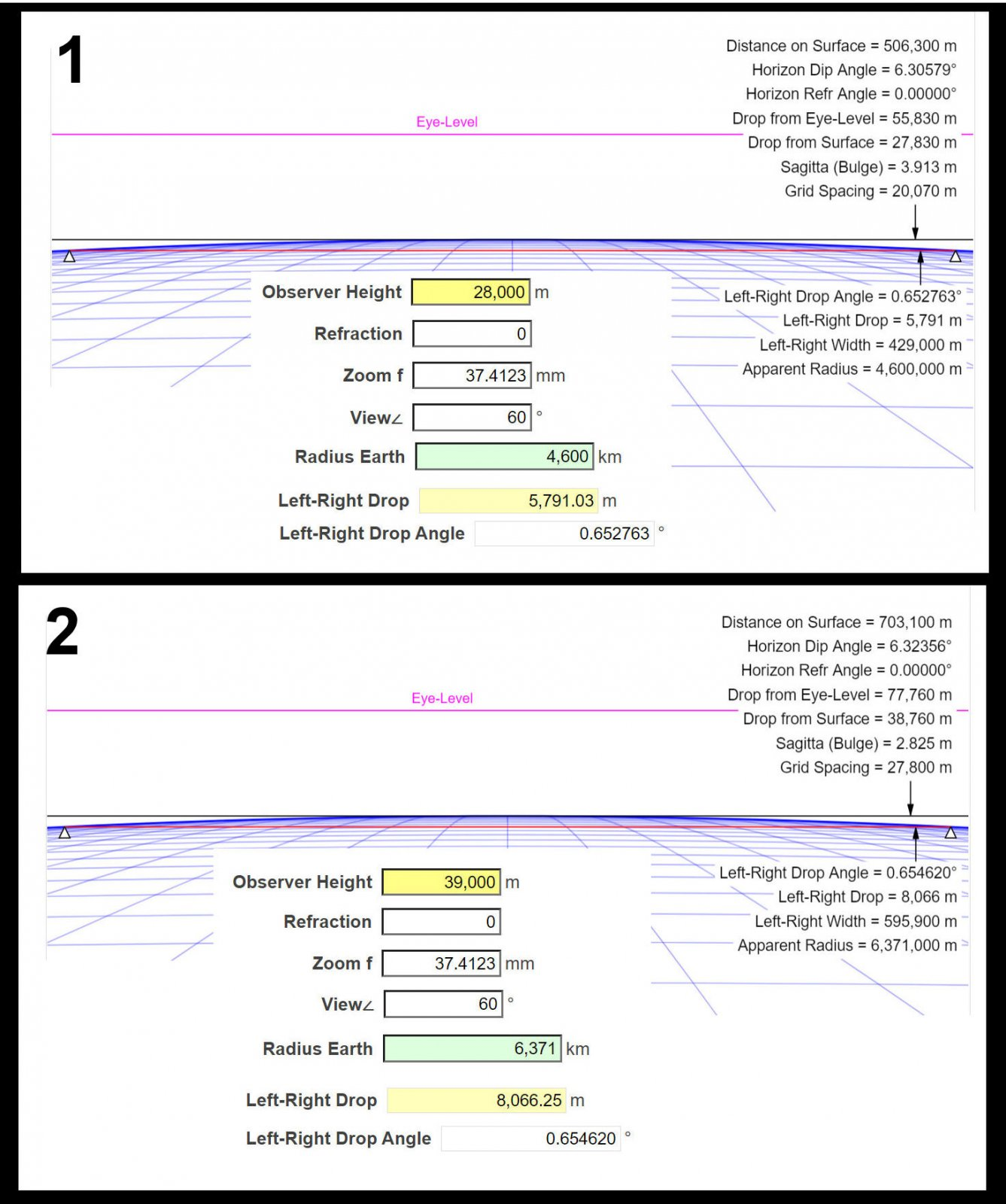The curves 1 and 2 look the same (and practically are). They represent the curvature of the horizon circle when h = 28 cm and the Miniearth's radius = 46 m. But the radius of the horizon circle is HorDistX d = 5.05 m.

Phil said: "The curve on the paper is the curve of the track. h is 28cm." The curve of the track must mean the circle with radius 46 m. But the curve on the paper is the circle with radius 5.05 m. So Phil really speaks about some other matter.

#### Rory

##### Closed Account
Does it matter that a running track isn't a circle?

I guess he means this bit in green: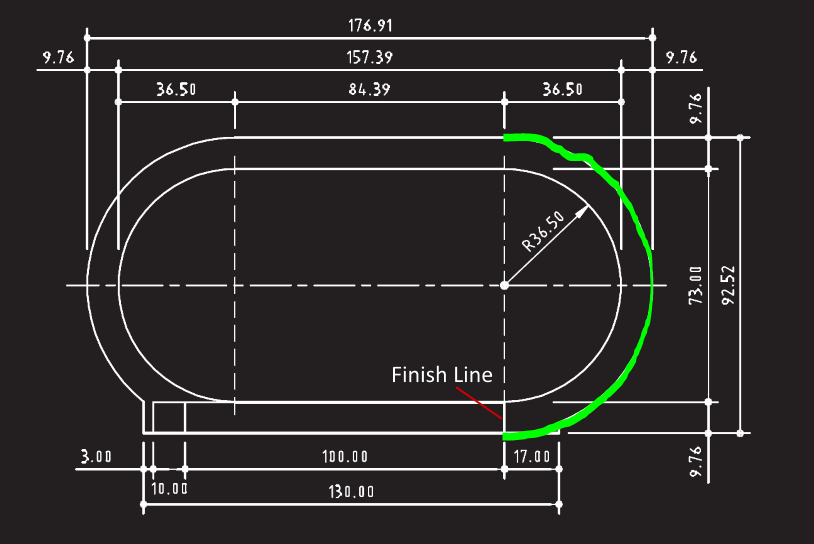A semi-circle with radius of 46.26m. Got it.

Last edited:

#### Mick West

Staff member
Again, you have to be careful about what you are actually measuring. It's a tricky thing. We see that the horizon seems to curve down to the left and the right in photos from high altitude, but the horizon isn't curving down, it's a circle, which is flat. It looks the same in every direction.

The idea with the racetrack (or my favorite, a circus ring) is to make this clear. We are looking at a circle from a point above the center of that circle. So we can divide the problem up into two parts.
1 - What's the radius of the circle, and how far above the plane of that circle are we.
2 - What does the circle look like from our position.

In the previous post (https://www.metabunk.org/threads/earth-curvature-simulation-by-walter-bislins.8986/post-274628) I described step 1, finding the values I labeled Z (the radius of the circle) and H (the height of the camera above the plane of the circle). Given those two numbers, and a field of view, you can do a rectilinear projection to get what it looks like.

Here's a circle, flat, seen from above: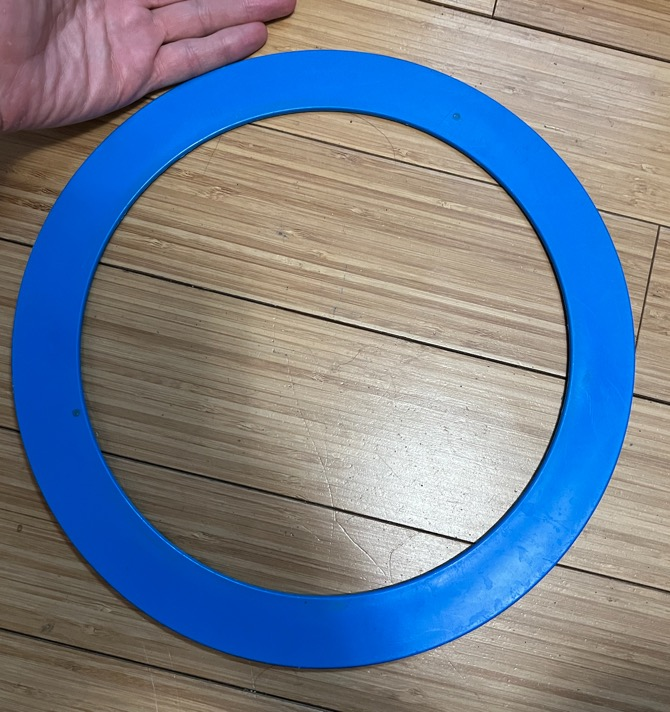And here is what it looks like from a point just above the center.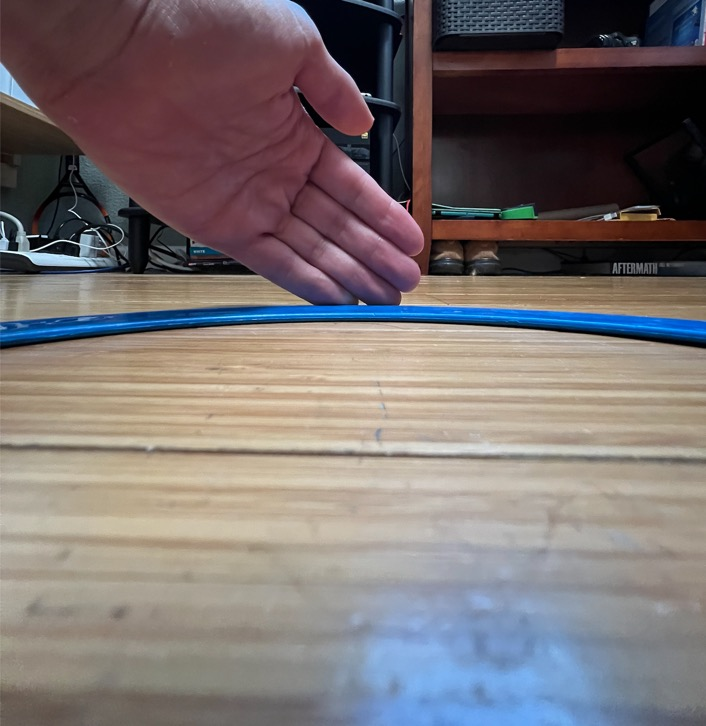Note the straight lines in the image, indicating this is essentially a rectilinear projection.

Putting a number on the drop at the sides is a bit tricky, as the angular size of a pixel varies with distance from the center of the image. Hence the two equations.

You could frame the problem in a different way, as the angle between the two vectors from the camera to these two 3D points: (circled on the left)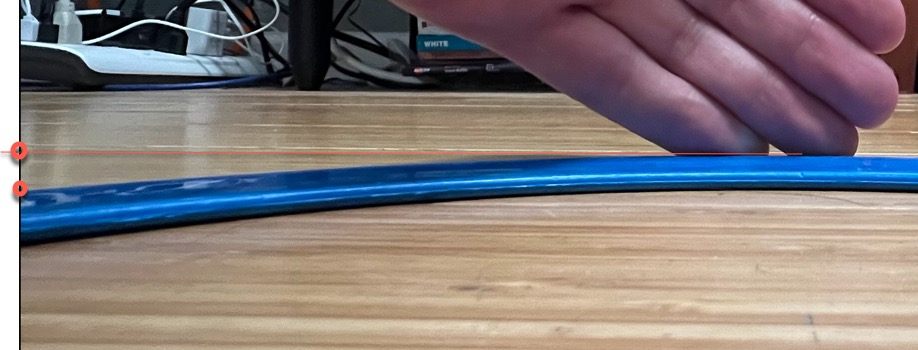But then the question becomes what are those two points? The bottom one is the intersection of the circle with the left plane of the view frustum. The top one is the intersection of the horizon plane with that frustum. That gives you a pure angular distance between the points in the image.

#### Pertti Niukkanen

##### Member
I guess he means this bit in green:
A semi-circle with radius of 46.26m. Got it.

The semicircle of a running track can be used in demonstrating the curvature of the horizon. The observer is at the centre of the circle looking the curving track at precise the correct height. Otherwise the track circle doesn't correspond to the horizon circle.

With curve calculator we find that at height of 166 m the distance to the horizon is 46 km. Scaling the figures by dividing by 1000 we get: Height of observer 16.6 cm, distance of horizon 46 m and radius of demo-Earth 6.37 km.

At small distances the height AB and sagitta BC are practically the same. So the height of observers eye must be 2*16.6 cm = 33.2 cm. Formula (1) gives at the field of view of 60 degrees the left-right drop angle δ = 0.06399 degrees = 3.84 arc minutes. Do you think you can see the curve?

One more time about Phil's example. Phil: "Outside of a 400m running track is ~46m. Scaling the earth down to that size puts Felix's jump at just over 28cm."

The height 28 cm is to scale with Earth's radius of 46 m. So Phil must think the situation like in the picture. The globe is cut in half and Felix is in the center. In miniworld his height is 28 cm and "normal" world 39 km. In both cases he can see on his flat Earth the horizon and its curvature too. What might be the angle δ now? Interesting question but not much to do with the curvature of the Globe.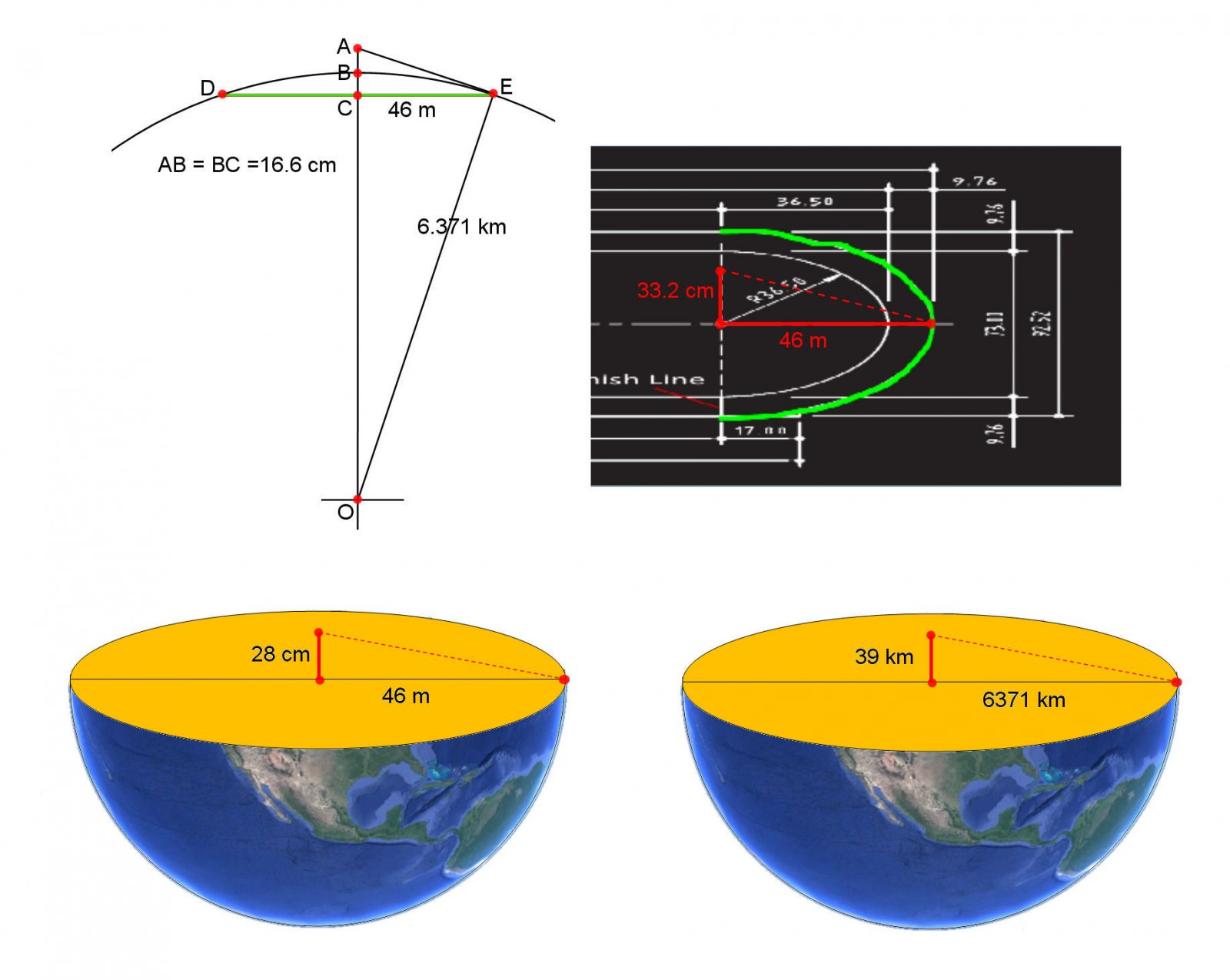#### Pertti Niukkanen

##### Member
What is the "left-right drop angle" δ on a flat Earth?

If R is the radius of the disk, and h the observer's height above the center of the disk, you can calculate the "left-right drop angle" δ of the horizon in a given field of view α.

One question may arise: What is the horizon in the FE model? It must be the edge of the disk. What else could it be?

I made the calculation in the case α = 60°. You can choose the radius R (though in my sketch the disk is the "standard" flat Earth model where the radius is 20000 km).

In the picture below is the derivation of formula (2). You can put the formula in Excel:
Select the cell C2 and type the formula

=(ATAN(A2/B2)-ACOS((2*B2)/NELIÖJUURI(3*(A2^2-B2^2))))*180/PII()

In English version replace PII() -> PI() and NELIÖJUURI -> SQRT

Some examples. Keeping the height constant h = 10, we get
R –––––– δ (deg)
20 ––––– 15.245
50 ––––– 2.3231
100 –––– 0.9537
500 –––– 0.1778
1000 ––– 0.0887

Keeping the radius constant R = 20000, we get
h –––––– δ (deg)
10 ––––– 0.00443
20 ––––– 0.00886
39 ––––– 0.0173
100 –––– 0.0443
1000 ––– 0.4516
2000 ––– 0.9537
5000 ––– 3.3098

The latter figures correspond to the flat Earth model. For example Felix B. would see on his jump The Edge curving by δ = 0.0173 degrees = 1.04 arc minutes.

Here the angle δ is calculated only above "the North Pole". So δ is the same in all directions. Looking in some other location must give different values in different directions. That's what I guess but I'm not sure. The drop of the horizon is certainly different.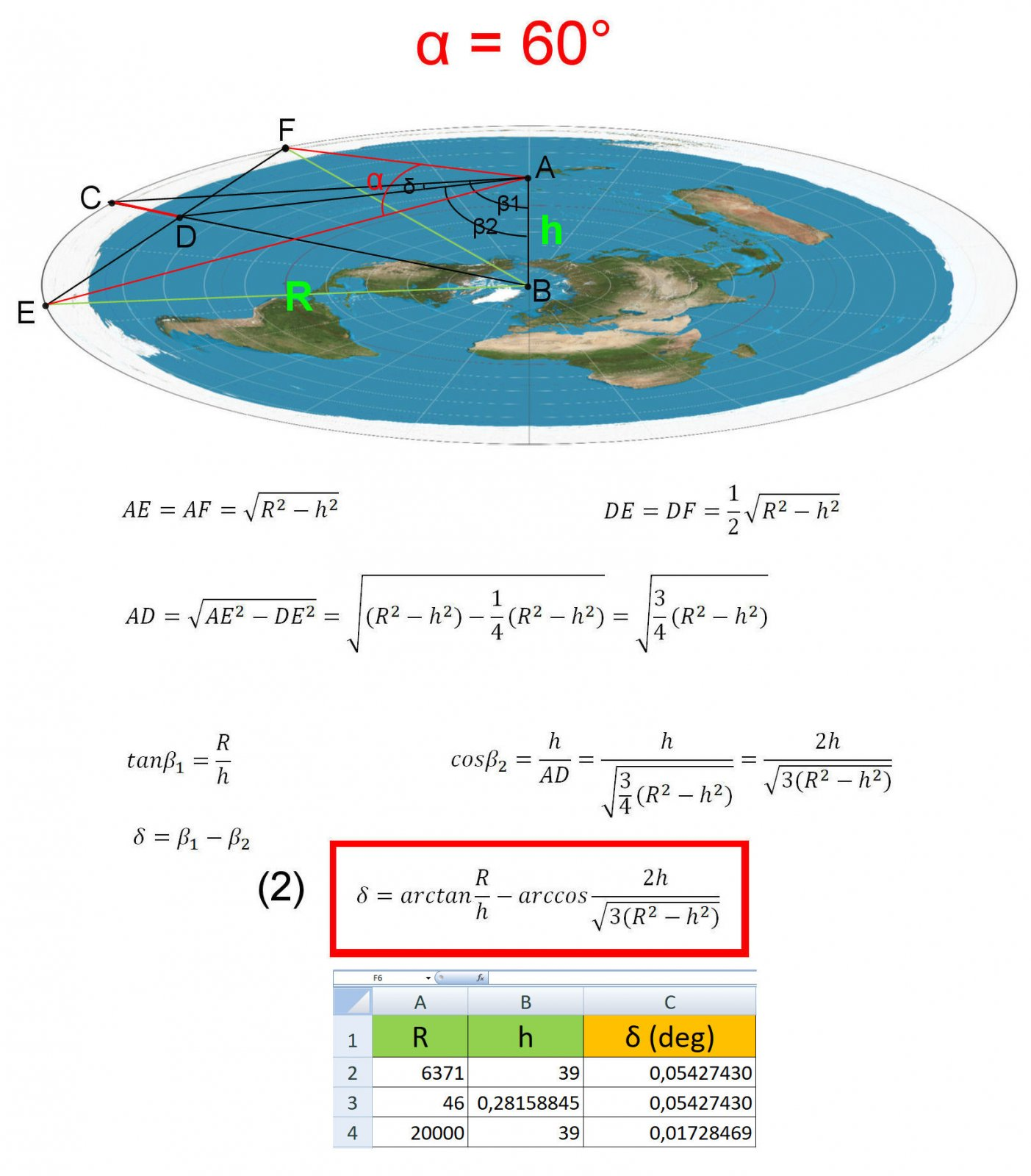#### Rory

##### Closed Account
One question may arise: What is the horizon in the FE model? It must be the edge of the disk. What else could it be?

While we know there wouldn't be a horizon on a flat earth, if you're going to work out something for their hypothetical model I guess you also have to use their hypothetical logic, where there is a horizon (no matter how peculiar the reasons).

#### Mick West

Staff member
Maybe I do not know how to use the equations Bislin gave. Would somebody please calculate the angle δ with Bislin's formulas (in situation like below)? Is the angle 0.1046 degrees (like in the picture) or 0.1571 degrees (like I got with the formulas)? Or maybe neither?
Bislins distinguishes between diagonal FOV and horizontal FOV, mixing these up may be a source of error?

And indeed it is. Although there seems to be some pitfalls here. It's helpful if we consider the simplest case of a camera with a square aspect ratio, 1:1
http://walter.bislins.ch/bloge/index.asp?page=Finding+the+curvature+of+the+Earth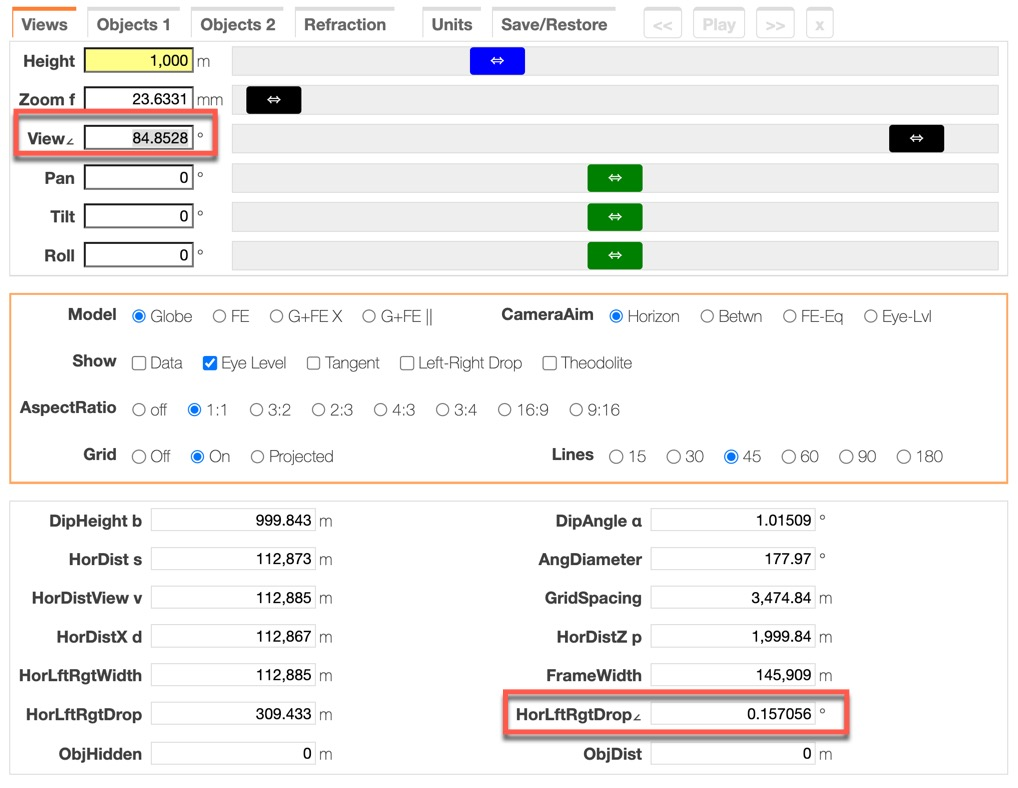84.8528? That's 60 * sqrt(2), it's simply the Pythagorean taking of the horizontal FOV, and converting it into the diagonal FOV, which is what the calculator expects. The result for the left/right drop angle is 0.157056

So that explains the discrepancy in the numbers. The pitfall here is that 84.8528 is NOT the diagonal FOV of a 60° wide square view. While the relationship between pixel distances is 1:1*sqrt(2), the angles don't scale linearly. To get the diagonal FOV from the horizontal FOV for a square aspect ratio, it's actually:

dfov = 2*atan(sqrt(2)*tan(hfov/2))

2*atan(sqrt(2)*tan(60/2 degrees)) in degrees = 78.46 degrees. (vs. 84.85). In Walter's calculator I think this only amounts to a quirk in inputting the FOV.

#### Pertti Niukkanen

##### Member
And indeed it is. Although there seems to be some pitfalls here. It's helpful if we consider the simplest case of a camera with a square aspect ratio, 1:1
http://walter.bislins.ch/bloge/index.asp?page=Finding+the+curvature+of+the+Earth

84.8528? That's 60 * sqrt(2), it's simply the Pythagorean taking of the horizontal FOV, and converting it into the diagonal FOV, which is what the calculator expects. The result for the left/right drop angle is 0.157056

So that explains the discrepancy in the numbers. The pitfall here is that 84.8528 is NOT the diagonal FOV of a 60° wide square view. While the relationship between pixel distances is 1:1*sqrt(2), the angles don't scale linearly. To get the diagonal FOV from the horizontal FOV for a square aspect ratio, it's actually:

dfov = 2*atan(sqrt(2)*tan(hfov/2))

2*atan(sqrt(2)*tan(60/2 degrees)) in degrees = 78.46 degrees. (vs. 84.85). In Walter's calculator I think this only amounts to a quirk in inputting the FOV.

Thank you for the efforts to find out the pitfalls in Walter's calculator. It was a great help to me.

I take these questions like some high school math/geometry/trig exercise. The pictures are just sketches helping comprehend the problem. The angular size of some geometric element is an angle in some triangle. I can understand the term "field of view" only in this sense: It is the angle at which some geometric element of the object is seen.

This is exactly what Walter did in the derivation of formula (1). There is only one correct answer and I expected to see it in the calculator. Now I can see it, thanks to you.

I understand that the pure geometric solution is not enough in analysing photographs. That is a subject I know nothing about, nor do I about cameras. Terms like diagonal FOV or horizontal FOV or pixel scaling are fussy to me. So I'm afraid your reasoning about pitfalls in Walter's calculator is like "caviar to the general" to me. But others like Mendel will surely benefit from it.

I still have some (maybe stupid) questions.

In the picture the height is 300000 m (In my mind was the height of ISS space station. Too late I found it's actually over 400 km). I want to know the left-right drop angle δ if the field of view is 40 degrees. So I put the values as in the picture below: View∠ = 40*sqrt(2) = 56.5685 and AspectRatio 1:1. Now the calculator gives HorLftRgtDrop∠ = 1.14528°. This is exactly the same as the value δ given by formula (1). So I'm very happy.

What about the curve picture then? Now I see a square delimiting the whole of view. The square comes certainly from AspectRatio 1:1 but how should I understand the view and drop angles?

Does the line segment a correspond to the angle of view = 56.5685° and the line segment b to the angle of view = 40°? It's fanny that the ratio a/b is about 1.5 like in my previous comparison with HorLftRgtDrop∠ in the calculator and the angle δ in formula (1). Is there some connection?

I think the value HorLftRgtDrop∠ = 1.14528° now corresponds to the square picture. When I choose Show Data and Left-Right Drop I get the picture on the right. Seems that the red line segment from which the drop is calculated doesn’t reach over the entire square. Is this only an inaccuracy in the drawing?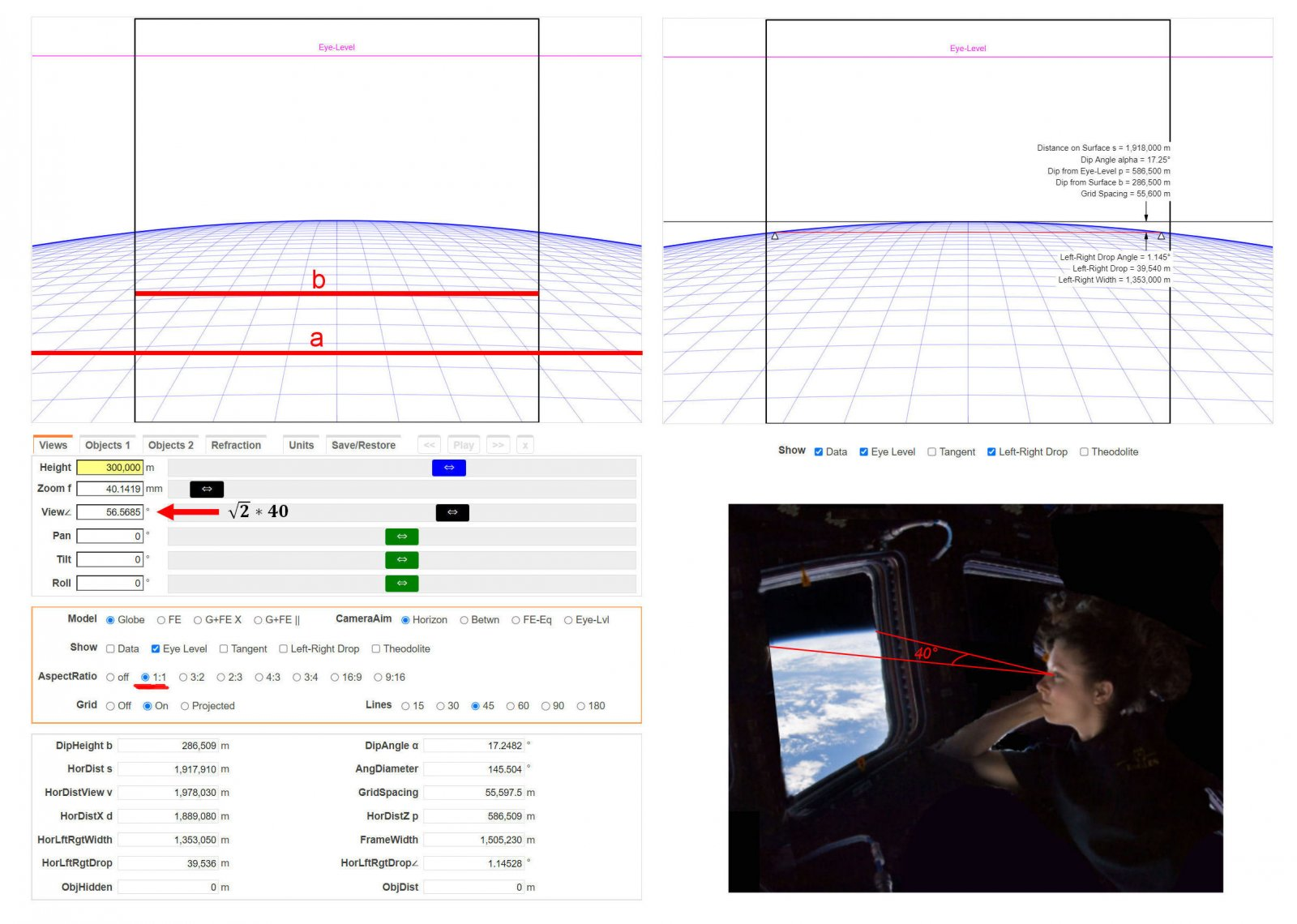The last picture is a clumsy photoshoped version of a photo where ISS-astronaut Tracy Caldwell Dyson is observing the Earth below. (https://commons.wikimedia.org/wiki/File:Tracy_Caldwell_Dyson_in_Cupola_ISS.jpg).

Let's think the window is a square and the glass makes no distortions (as if it's nonexistent). Tracy's horizontal angle of view is 40°. Is it now proper to say that the square seen in the calculator corresponds to what she can see through the window?

#### Mick West

Staff member
I understand that the pure geometric solution is not enough in analysing photographs. That is a subject I know nothing about, nor do I about cameras. Terms like diagonal FOV or horizontal FOV or pixel scaling are fussy to me.
[Field of View] is the angle at which some geometric element of the object is seen.
Think of the eye, or the camera, as being in the middle of a scene, like it's in the middle of a giant sphere.

For any point on any object in the scene, you can draw a line from that point to the camera. This is called a Line of Sight (LOS).

For any two points in the scene there are exactly two lines of sight, going to the camera (or the eye).

We can measure the angle between any two lines of sight. This is often called the "visual angle", or the "angle subtended at the eye", or the "angular size" (when the two points are the extents of the object).

Importantly: this is entirely independent of the Field of View of the camera, or the eye.

Field of view is just the angle between a point just visible on one side of the camera (real or virtual), and a point just visible on the other side of the camera. Typically this is given as the horizontal extent, but can also be diagonal, or vertical (the software I use for Sitrec uses vertical).

A photo is flat. How we go from the real world to a flat surface is "projection" - which is literally like what it sounds like, projecting light onto a flat surface, like a movie projector projects an image onto a screen. Most photos use "rectilinear" projection (aka perspective projection), where, essentially the LOS just go straight through a focal point (the optical center) and then hit the flat sensor (or film)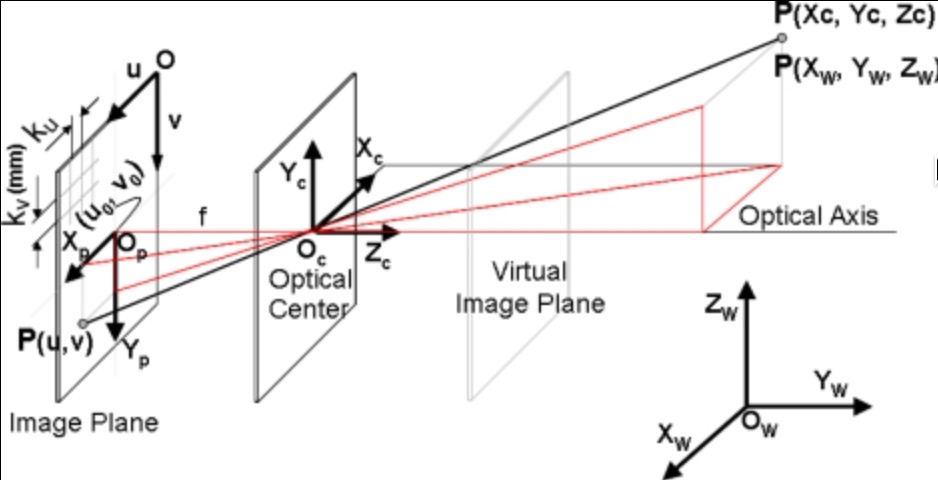The sensor (the image plane) is behind the focal point in a camera, so you get an inverted image.In computer graphics it's much simpler to think of in terms of the "virtual image plane", and put that in front of the virtual camera.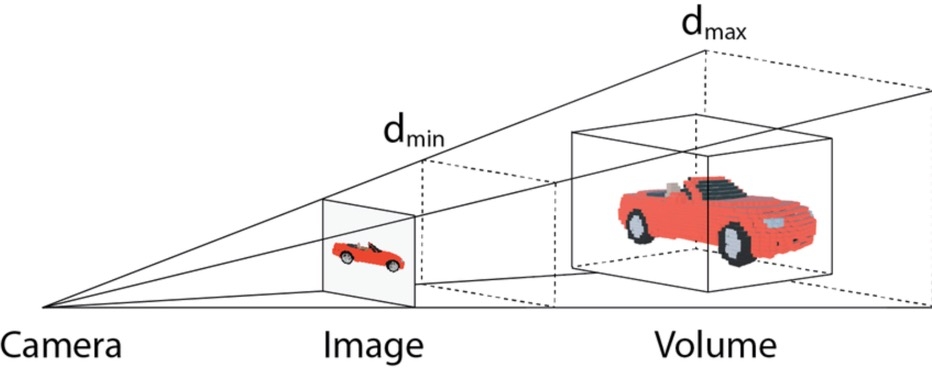This is much easier to visualize, no image flipping, and fewer lines. Mathematically it's really the same.

So if we've got a 60° horizontal FOV, the angle between the center of the image and the edges is 30°, and it's tempting to think that the angle between the center and half-way to the edge is then 15*. But it's not, it's 16.1 degrees (atan(tan(30)/2))